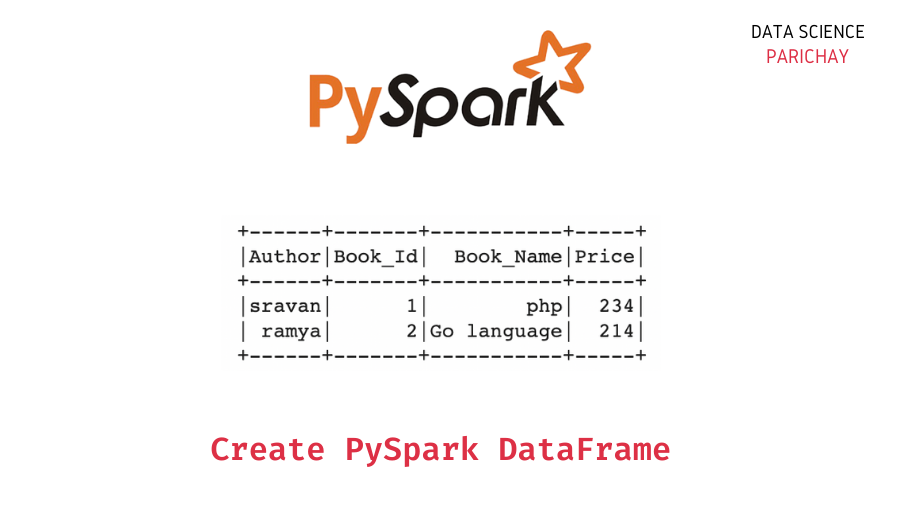# Create a Dataframe in Pyspark

In this article, we will discuss PySpark and how to create a DataFrame in PySpark with the help of some examples.

## Spark

Spark is a big data framework used to store and process huge amounts of data.

• Using Spark we can create, update and delete the data. It has a large memory and processes the data multiple times faster than the normal computing system.
• It supports Java, Scala, and Python languages.

### Features of Spark

• Faster – It is faster in terms of computing and accessibility.
• Scalable – We can extend our application from single to bulk in terms of processing,
inserting, and updating the data.

### PySpark

PySpark is a module in Python used to store and process the data with the Spark framework.

To use PySpark we have to install the PySpark module in our local machine using the command `pip`.

`pip install pyspark`

Then, we have to create our Spark app after installing the module. The following are the steps to create a spark app in Python.

STEP 1 – Import the `SparkSession` class from the SQL module through PySpark

`from pyspark.sql import SparkSession`

Step 2 – Create a Spark app using the `getOrcreate()` method. The following is the syntax –

`spark = SparkSession.builder.appName('sample_spark_app').getOrCreate()`

This way we can create our own Spark app through PySpark in Python.

📚 Data Science Programs By Skill Level

Introductory

Intermediate ⭐⭐⭐

🔎 Find Data Science Programs 👨‍💻 111,889 already enrolled

Disclaimer: Data Science Parichay is reader supported. When you purchase a course through a link on this site, we may earn a small commission at no additional cost to you. Earned commissions help support this website and its team of writers.

Now let’s use this Spark app to create a PySpark DataFrame. We can create a PySpark dataframe using the `createDataFrame()` method. The following is the syntax –

`spark.createDataFrame(DataFrame, [columns])`

Here “DataFrame” is the input dataframe and “columns” are the column names in the dataframe to be provided.

### Examples

Let’s look at some examples of using the above syntax to create a Pyspark dataframe.

Example 1 – PySpark dataframe from a list of lists.

```#import the pyspark module
import pyspark

# import the  sparksession class  from pyspark.sql
from pyspark.sql import SparkSession

# create an app from SparkSession class
spark = SparkSession.builder.appName('my_spark_app').getOrCreate()

# books data as list of lists
df = [[1, "php", "sravan", 234],
[2, "sql", "chandra sekhar", 345],
[3, "python", "harsha", 1200],
[4, "R", "Rohith", 120],
]

# creating a dataframe from the books data by specifying the columns
dataframe = spark.createDataFrame(df, ['Book_Id', 'Book_Name', 'Author', 'Price'])

#display
dataframe.show()```

Output:

```+-------+---------+--------------+-----+
|Book_Id|Book_Name|        Author|Price|
+-------+---------+--------------+-----+
|      1|      php|        sravan|  234|
|      2|      sql|chandra sekhar|  345|
|      3|   python|        harsha| 1200|
|      4|        R|        Rohith|  120|
+-------+---------+--------------+-----+```

Example 2 – PySpark dataframe from a list of tuples. The code is very similar to the one used in the example above.

```#import the pyspark module
import pyspark

# import the  sparksession class  from pyspark.sql
from pyspark.sql import SparkSession

# create an app from SparkSession class
spark = SparkSession.builder.appName('my_spark_app').getOrCreate()

# books data as list of tuples
df = [(1, "php", "sravan", 234),
(2, "sql", "chandra sekhar", 345),
(3, "python", "harsha", 1200),
(4, "R", "Rohith", 120),
]

# create a dataframe from the books data by specifying the columns
dataframe = spark.createDataFrame(df, ['Book_Id', 'Book_Name', 'Author', 'Price'])

#display
dataframe.show()```

Output:

```+-------+---------+--------------+-----+
|Book_Id|Book_Name|        Author|Price|
+-------+---------+--------------+-----+
|      1|      php|        sravan|  234|
|      2|      sql|chandra sekhar|  345|
|      3|   python|        harsha| 1200|
|      4|        R|        Rohith|  120|
+-------+---------+--------------+-----+```

Example 3 – PySpark dataframe from a list of dictionaries.

```#import the pyspark module
import pyspark

# import the  sparksession class  from pyspark.sql
from pyspark.sql import SparkSession

# create an app from SparkSession class
spark = SparkSession.builder.appName('my_spark_app').getOrCreate()

# books data as list of dictionaries
df = [{'Book_Id':1, 'Book_Name':"php",'Author' :"sravan",'Price': 234},
{'Book_Id':2, 'Book_Name':"Go language",'Author' :"ramya",'Price': 214},
]

# creating a dataframe from the books data (list of dictinaries)
dataframe = spark.createDataFrame(df)

#display
dataframe.show()```

Output:

```+------+-------+-----------+-----+
|Author|Book_Id|  Book_Name|Price|
+------+-------+-----------+-----+
|sravan|      1|        php|  234|
| ramya|      2|Go language|  214|
+------+-------+-----------+-----+```

You can see that here we didn’t need to specify the column names in the `createDataFrame()` function like we did in the above examples.

You might also be interested in –

••